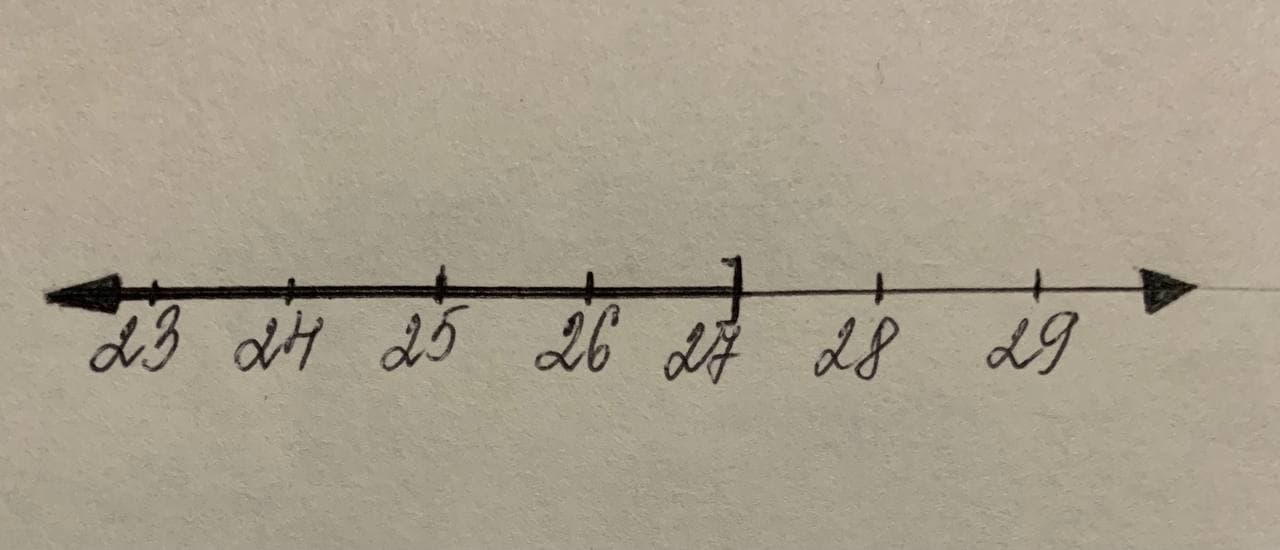# To calculate: The solution set of the compound inequality -4\le-\frac{2}{3}p+14,pavitorj6 2021-11-21 Answered
To calculate: The solution set of the compound inequality $$\displaystyle-{4}\le-{\frac{{{2}}}{{{3}}}}{p}+{14}$$, graph it, and write it in set-builder notation and interval notation.

• Questions are typically answered in as fast as 30 minutes

### Plainmath recommends

• Get a detailed answer even on the hardest topics.
• Ask an expert for a step-by-step guidance to learn to do it yourself.navratitehf
Given Information:
The compound inequality, $$\displaystyle-{4}\le-{\frac{{{2}}}{{{3}}}}{p}+{14}$$
Calculation:
The given compound inequality is $$\displaystyle-{4}\le-{\frac{{{2}}}{{{3}}}}{p}+{14}$$. Subtract 14 from the inequality.
$$\displaystyle-{4}-{14}\le-{\frac{{{2}}}{{{3}}}}{p}+{14}-{14}$$
$$\displaystyle-{18}\le-{\frac{{{2}}}{{{3}}}}{p}$$
Divide the inequality by $$\displaystyle-{\frac{{{2}}}{{{3}}}}$$ and change the sense of inequality,
$$\displaystyle{\frac{{-{18}}}{{{\left(-{\frac{{{2}}}{{{3}}}}\right)}}}}\geq{\frac{{-{\frac{{{2}}}{{{3}}}}}}{{{\left(-{\frac{{{2}}}{{{3}}}}\right)}}}}{p}$$
After division,
$$\displaystyle-{18}\times-{\frac{{{3}}}{{{2}}}}\geq-{\frac{{{2}}}{{{3}}}}\times-{\frac{{{3}}}{{{2}}}}{p}$$
$$\displaystyle{27}\geq{p}$$
The graph of the solution is:Therefore, the solution set of compound inequality $$\displaystyle-{4}\le-{\frac{{{2}}}{{{3}}}}{p}+{14}$$ is $$\displaystyle{\left\lbrace{p}{\mid}{p}\le{27}\right\rbrace}$$ and interval notation is $$\displaystyle{\left(-\propto,{27}\right]}$$.